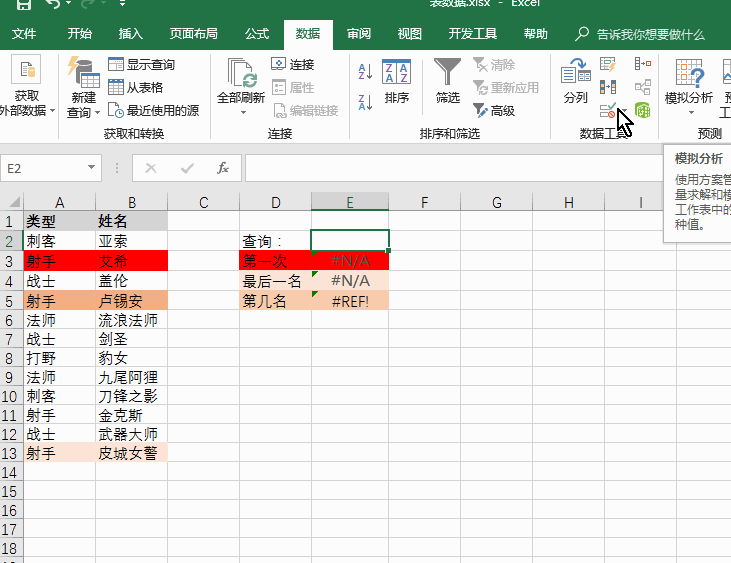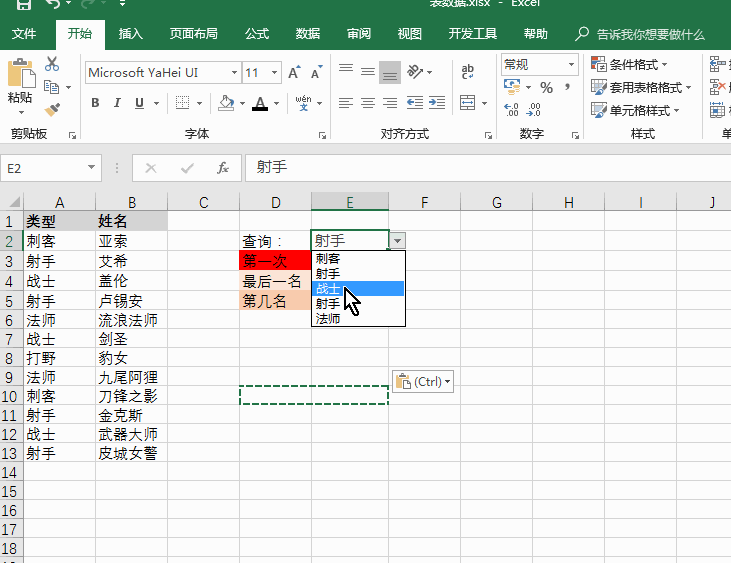# Excel快速查找三剑客

=INDEX(区域,SMALL(IF(条件,行号数组,4^8),ROW(A1)))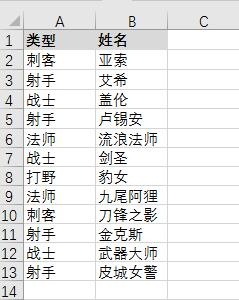1，射手第一次出现时的B列值；

2，射手最后一次出现时的B列值；

3，射手第n次出现时候的B列值（假设n=2）。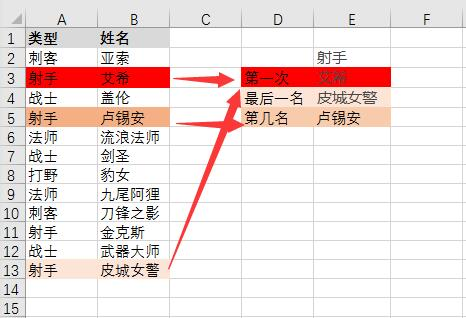=VLOOKUP(E2,A2:B13,2,FALSE)

=LOOKUP(1,0/(A2:A13=E2),B2:B13)

{=INDEX(B\$2:B\$13,SMALL(IF(A\$2:A\$13=E\$2,ROW(\$1:\$12),4^8),2))}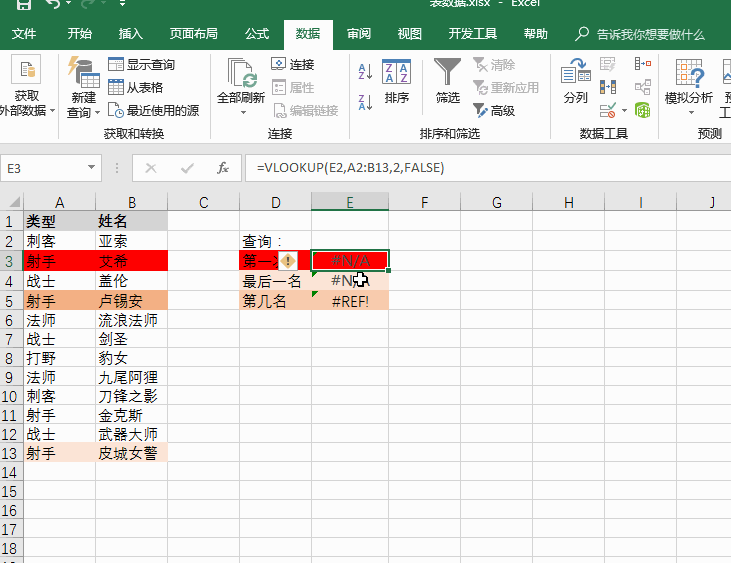IF(A\$2:A\$13=E\$2,ROW(\$1:\$12),4^8)

{1;65536;……;65536;12;13;65536;65536;65536}